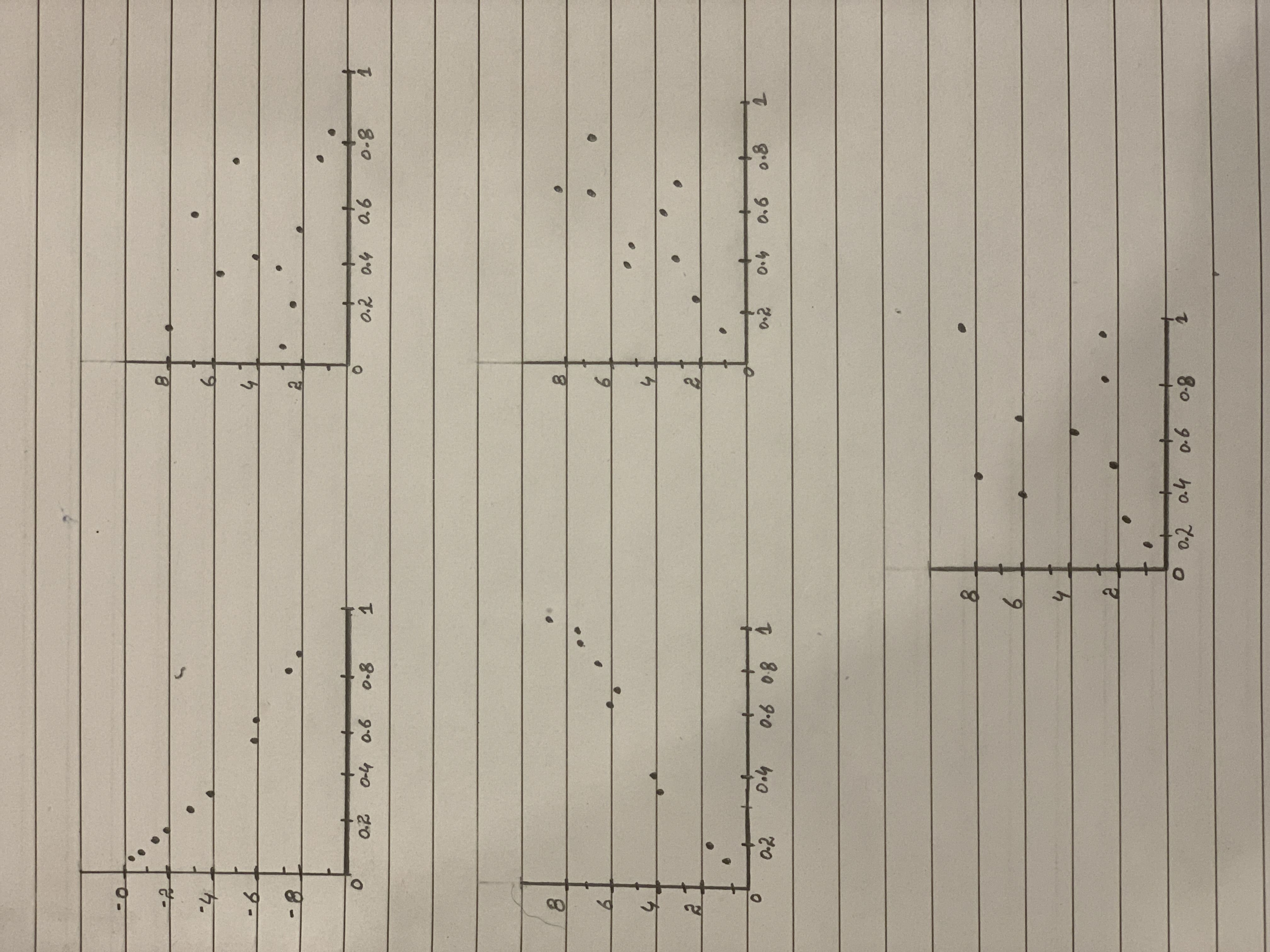# Match the values of r with the accompanying scatterplots 0.723,-0.353,0.353,0.998 and -0.998scatterplots 1,2,3,4,5<img src="13310101111.JPG">babeeb0oL 2021-03-05 Answered

Match the values of r with the accompanying scatterplots 0.723,-0.353,0.353,0.998 and -0.998
scatterplots 1,2,3,4,5You can still ask an expert for help

• Questions are typically answered in as fast as 30 minutes

Solve your problem for the price of one coffee

• Math expert for every subject
• Pay only if we can solve itJaylen Fountain
Correlation coefficient:
The value of correlation coefficient (r) ranges from –1 to 1.
The value of r closer to –1 or 1 indicates that there is strong correlation between two quantitative variables, whereas, the value of r closer to 0 indicates that there is weak or no correlation between variables.
The positive value of correlation indicates that both the variables (say x and y) are in same direction, whereas, the negative value of correlation indicates that both the variables are in opposite direction.
Match the following:
In scatter plot 1, the variables x and y are in opposite direction and it is approximately linear. Therefore, it has highest negative correlation. Among the given correlation values, it is clear that the r value for the scatter plot 1 is –0.998.
Similarly, by comparing the scatter plots with the r values, the scatterplots are matched with their respective r values as shown below:
Scatterplot 1, r = –0.998
Scatterplot 2, r = –0.353
Scatterplot 3, r = 0.998
Scatterplot 4, r = 0.723
Scatterplot 5, r = 0.353